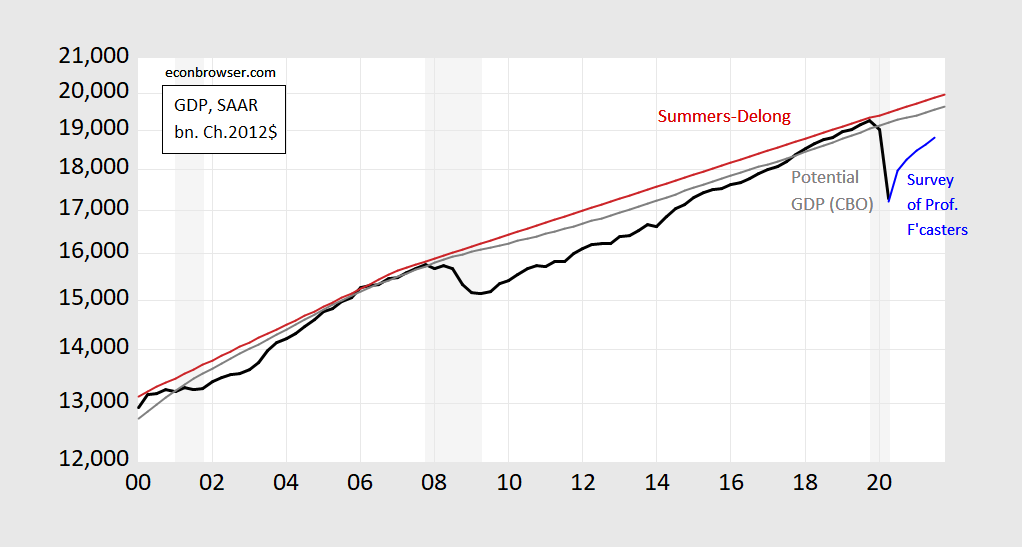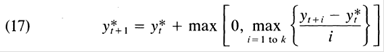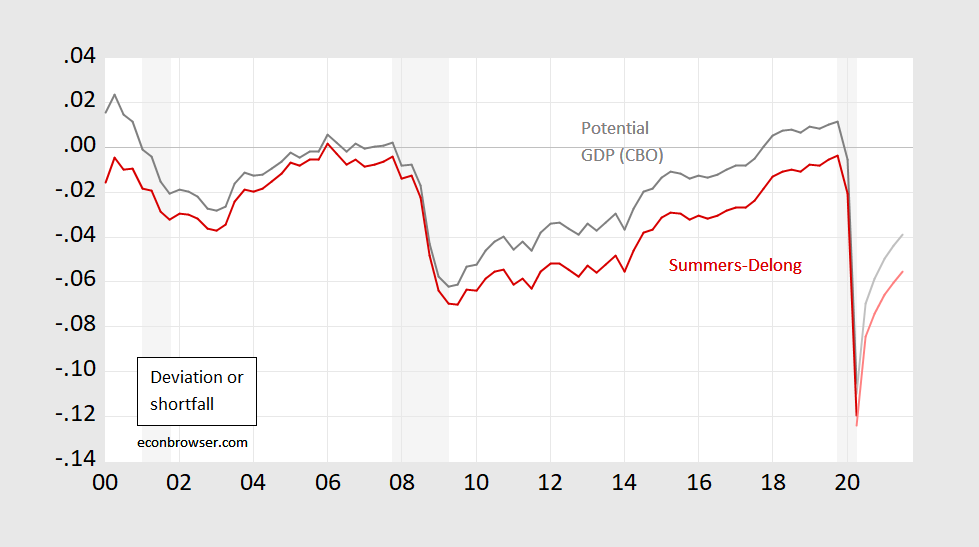### Deviations vs. ShortfallsThe Fed’s new framework, as described by Chairman Powell, mentions “shortfalls” (particularly in employment), instead of deviations of the natural rate. The output analog of this shift is moving from the deviation of output from potential (i.e., output gap) to an output slack measure. If we interpret this as requiring a focus on a Friedman-esque plucking model of maximal output, rather than potential GDP as described in most textbooks, what does this mean for where we are right now? I’d say for the short run, we are still in for a world of pain, economically speaking…

Following the description in this post (see also this post), I implement a Summers-Delong (BPEA, 1988) measure of maximal output.Figure 1: GDP (bold black), August Survey of Professional Forecasters mean forecast (light blue), CBO potential GDP (gray), pseudo-Summers-Delong (k=5) using actual data and CBO projections of GDP to 2030 (red). NBER recession dates shaded gray; assumes latest recession ends 2020Q2. Source: BEA, CBO An Update of the Economic Outlook (July 2020), NBER, and author’s calculations.

The formula used is given by recursive application of Summers and Delong (1988) equation 17:Where y* is potential GDP, and k=3 to 5. I calculate using annual data, then interpolate using quadratic match to quarterly frequency.

This implies the following for (log) output gap or shortfall:Figure 2: Log output gap using potential GDP (gray), or log shortfall pseudo-Summers-Delong (k=5) using actual data and CBO projections of GDP to 2030 (red). Q3 data uses August Survey of Professional Forecasters mean forecast. NBER recession dates shaded gray; assumes latest recession ends 2020Q2. Source: BEA, CBO An Update of the Economic Outlook (July 2020), NBER, and author’s calculations.

For the moment, economic activity looks very down regardless of measure. In Q2, it’s 10.5% vs 12% (conventional gap vs shortfall). Even after a forecasted jump in Q3, it’ll still be 6.9% vs. 8.4%